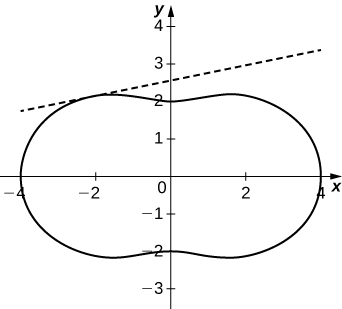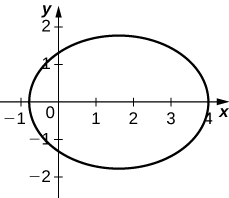$$\newcommand{\id}{\mathrm{id}}$$ $$\newcommand{\Span}{\mathrm{span}}$$ $$\newcommand{\kernel}{\mathrm{null}\,}$$ $$\newcommand{\range}{\mathrm{range}\,}$$ $$\newcommand{\RealPart}{\mathrm{Re}}$$ $$\newcommand{\ImaginaryPart}{\mathrm{Im}}$$ $$\newcommand{\Argument}{\mathrm{Arg}}$$ $$\newcommand{\norm}{\| #1 \|}$$ $$\newcommand{\inner}{\langle #1, #2 \rangle}$$ $$\newcommand{\Span}{\mathrm{span}}$$

# 4E: Chapter Exercises

$$\newcommand{\vecs}{\overset { \rightharpoonup} {\mathbf{#1}} }$$

$$\newcommand{\vecd}{\overset{-\!-\!\rightharpoonup}{\vphantom{a}\smash {#1}}}$$

## Chapter Review Exercises

True or False? Justify your answer with a proof or a counterexample.

1) The rectangular coordinates of the point $$\displaystyle (4,\frac{5π}{6})$$ are $$\displaystyle (2\sqrt{3},−2).$$

2) The equations $$\displaystyle x=cosh(3t), y=2sinh(3t)$$ represent a hyperbola.

Solution: True.

3) The arc length of the spiral given by $$\displaystyle r=\frac{θ}{2}$$ for $$\displaystyle 0≤θ≤3π$$ is $$\displaystyle \frac{9}{4}π^3$$.

4) Given $$\displaystyle x=f(t)$$ and $$\displaystyle y=g(t)$$, if $$\displaystyle \frac{dx}{dy}=\frac{dy}{dx}$$, then $$\displaystyle f(t)=g(t)+C,$$ where $$\displaystyle C$$ is a constant.

Solution: False. Imagine $$\displaystyle y=t+1, x=−t+1.$$

For the following exercises, sketch the parametric curve and eliminate the parameter to find the Cartesian equation of the curve.

5) $$\displaystyle x=1+t, y=t^2−1, −1≤t≤1$$

6) $$\displaystyle x=e^t, y=1−e^{3t}, 0≤t≤1$$

Solution: $$\displaystyle y=1−x^3$$7) $$\displaystyle x=sinθ, y=1−cscθ, 0≤θ≤2π$$

8) $$\displaystyle x=4cosϕ, y=1−sinϕ, 0≤ϕ≤2π$$

Solution: $$\displaystyle \frac{x^2}{16}+(y−1)^2=1$$For the following exercises, sketch the polar curve and determine what type of symmetry exists, if any.

9) $$\displaystyle r=4sin(\frac{θ}{3})$$

10) $$\displaystyle r=5cos(5θ)$$

Solution: Symmetric about polar axisFor the following exercises, find the polar equation for the curve given as a Cartesian equation.

11) $$\displaystyle x+y=5$$

12) $$\displaystyle y^2=4+x^2$$

Solution: $$\displaystyle r^2=\frac{4}{sin^2θ−cos^2θ}$$

For the following exercises, find the equation of the tangent line to the given curve. Graph both the function and its tangent line.

13) $$\displaystyle x=ln(t), y=t^2−1, t=1$$

14) $$\displaystyle r=3+cos(2θ), θ=\frac{3π}{4}$$

Solution: $$\displaystyle y=\frac{3\sqrt{2}}{2}+\frac{1}{5}(x+\frac{3\sqrt{2}}{2})$$15) Find $$\displaystyle \frac{dy}{dx}, \frac{dx}{dy},$$ and $$\displaystyle \frac{d^2x}{dy^2}$$ of $$\displaystyle y=(2+e^{−t}), x=1−sin(t)$$

For the following exercises, find the area of the region.

16) $$\displaystyle x=t^2, y=ln(t), 0≤t≤e$$

Solution: $$\displaystyle \frac{e^2}{2}$$

17) $$\displaystyle r=1−sinθ$$ in the first quadrant

For the following exercises, find the arc length of the curve over the given interval.

18) $$\displaystyle x=3t+4, y=9t−2, 0≤t≤3$$

Solution: $$\displaystyle 9\sqrt{10}$$

19) $$\displaystyle r=6cosθ, 0≤θ≤2π.$$ Check your answer by geometry.

For the following exercises, find the Cartesian equation describing the given shapes.

20) A parabola with focus $$\displaystyle (2,−5)$$ and directrix $$\displaystyle x=6$$

Solution: $$\displaystyle (y+5)^2=−8x+32$$

21) An ellipse with a major axis length of 10 and foci at $$\displaystyle (−7,2)$$ and $$\displaystyle (1,2)$$

22) A hyperbola with vertices at $$\displaystyle (3,−2)$$ and $$\displaystyle (−5,−2)$$ and foci at $$\displaystyle (−2,−6)$$ and $$\displaystyle (−2,4)$$

Solution: $$\displaystyle \frac{(y+1)^2}{16}−\frac{(x+2)^2}{9}=1$$

For the following exercises, determine the eccentricity and identify the conic. Sketch the conic.

23) $$\displaystyle r=\frac{6}{1+3cos(θ)}$$

24) $$\displaystyle r=\frac{4}{3−2cosθ}$$

Solution: $$\displaystyle e=\frac{2}{3}$$, ellipse25) $$\displaystyle r=\frac{7}{5−5cosθ}$$

26) Determine the Cartesian equation describing the orbit of Pluto, the most eccentric orbit around the Sun. The length of the major axis is 39.26 AU and minor axis is 38.07 AU. What is the eccentricity?

Solution: $$\displaystyle \frac{y^2}{19.03^2}+\frac{x^2}{19.63^2}=1, e=0.2447$$

27) The C/1980 E1 comet was observed in 1980. Given an eccentricity of 1.057 and a perihelion (point of closest approach to the Sun) of 3.364 AU, find the Cartesian equations describing the comet’s trajectory. Are we guaranteed to see this comet again? (Hint: Consider the Sun at point $$\displaystyle (0,0)$$.)